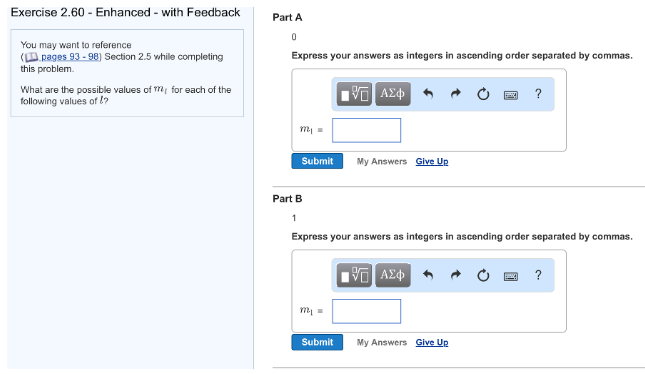# Problem: What are the possible values of ml for each of the following values of l? Part A0Express your answers as integers in ascending order separated by commas. Part B1 Express your answers as integers in ascending order separated by commas.

###### FREE Expert Solution
87% (76 ratings)###### Problem Details

What are the possible values of ml for each of the following values of l?

Part A

0

Part B